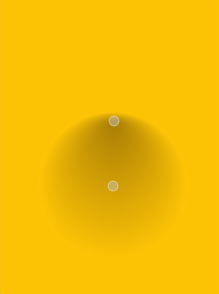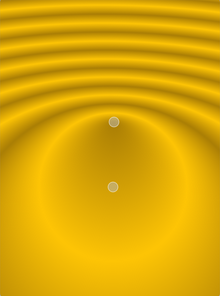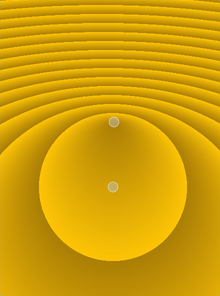The `QRadialGradient` class is used in combination with `QBrush` to specify a radial gradient brush. More

## Detailed Description¶

Qt supports both simple and extended radial gradients.

Simple radial gradients interpolate colors between a focal point and end points on a circle surrounding it. Extended radial gradients interpolate colors between a focal circle and a center circle. Points outside the cone defined by the two circles will be transparent. For simple radial gradients the focal point is adjusted to lie inside the center circle, whereas the focal point can have any position in an extended radial gradient.

Outside the end points the gradient is either padded, reflected or repeated depending on the currently set `spread` method:`PadSpread` (default) `ReflectSpread` `RepeatSpread`

The colors in a gradient is defined using stop points of the `QGradientStop` type, i.e. a position and a color. Use the `setColorAt()` or the `setStops()` function to define the stop points. It is the gradient’s complete set of stop points that describes how the gradient area should be filled. If no stop points have been specified, a gradient of black at 0 to white at 1 is used.

In addition to the functions inherited from `QGradient` , the `QRadialGradient` class provides the `center()` , `focalPoint()` and `radius()` functions returning the gradient’s center, focal point and radius respectively.

Parameters

Constructs a simple radial gradient with the center and focal point at (0, 0) with a radius of 1.

Constructs an extended radial gradient with the given `center`, `centerRadius`, `focalPoint`, and `focalRadius`.

Constructs a simple radial gradient with the given `center`, `radius` and the focal point in the circle center.

Constructs a simple radial gradient with the given `center`, `radius` and `focalPoint`.

Note

If the given focal point is outside the circle defined by the `center` point and `radius`, it will be re-adjusted to lie at a point on the circle where it intersects with the line from `center` to `focalPoint`.

Constructs an extended radial gradient with the given center (`cx`, `cy`), center radius, `centerRadius`, focal point, (`fx`, `fy`), and focal radius `focalRadius`.

Constructs a simple radial gradient with the center at (`cx`, `cy`) and the specified `radius`. The focal point lies at the center of the circle.

Constructs a simple radial gradient with the given center (`cx`, `cy`), `radius` and focal point (`fx`, `fy`).

Note

If the given focal point is outside the circle defined by the center (`cx`, `cy`) and the `radius` it will be re-adjusted to the intersection between the line from the center to the focal point and the circle.

Return type

`PySide6.QtCore.QPointF`

Returns the center of this radial gradient in logical coordinates.

Return type

float

Returns the center radius of this radial gradient in logical coordinates.

Return type

`PySide6.QtCore.QPointF`

Returns the focal point of this radial gradient in logical coordinates.

Return type

float

Returns the focal radius of this radial gradient in logical coordinates.

Return type

float

Returns the radius of this radial gradient in logical coordinates.

Equivalent to `centerRadius()`

Parameters

Sets the center of this radial gradient in logical coordinates to `center`.

Parameters
• x – float

• y – float

This is an overloaded function.

Sets the center of this radial gradient in logical coordinates to (`x`, `y`).

Parameters

Sets the center radius of this radial gradient in logical coordinates to `radius`

Parameters

focalPoint`PySide6.QtCore.QPointF`

Sets the focal point of this radial gradient in logical coordinates to `focalPoint`.

Parameters
• x – float

• y – float

This is an overloaded function.

Sets the focal point of this radial gradient in logical coordinates to (`x`, `y`).

Parameters

Sets the focal radius of this radial gradient in logical coordinates to `radius`
Sets the radius of this radial gradient in logical coordinates to `radius`
Equivalent to `setCenterRadius()`